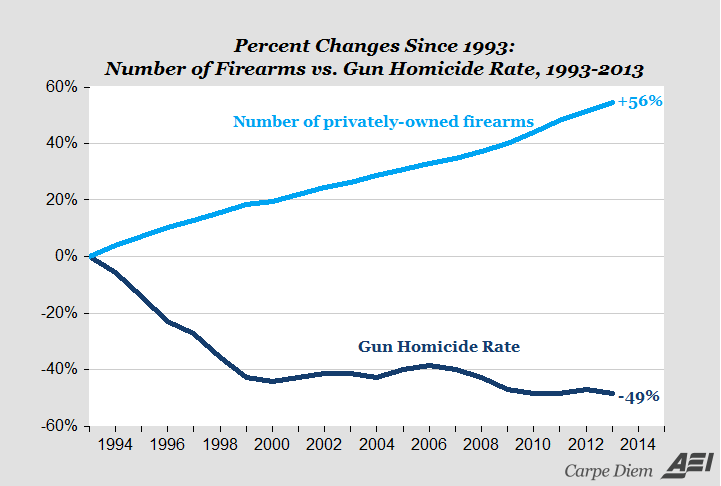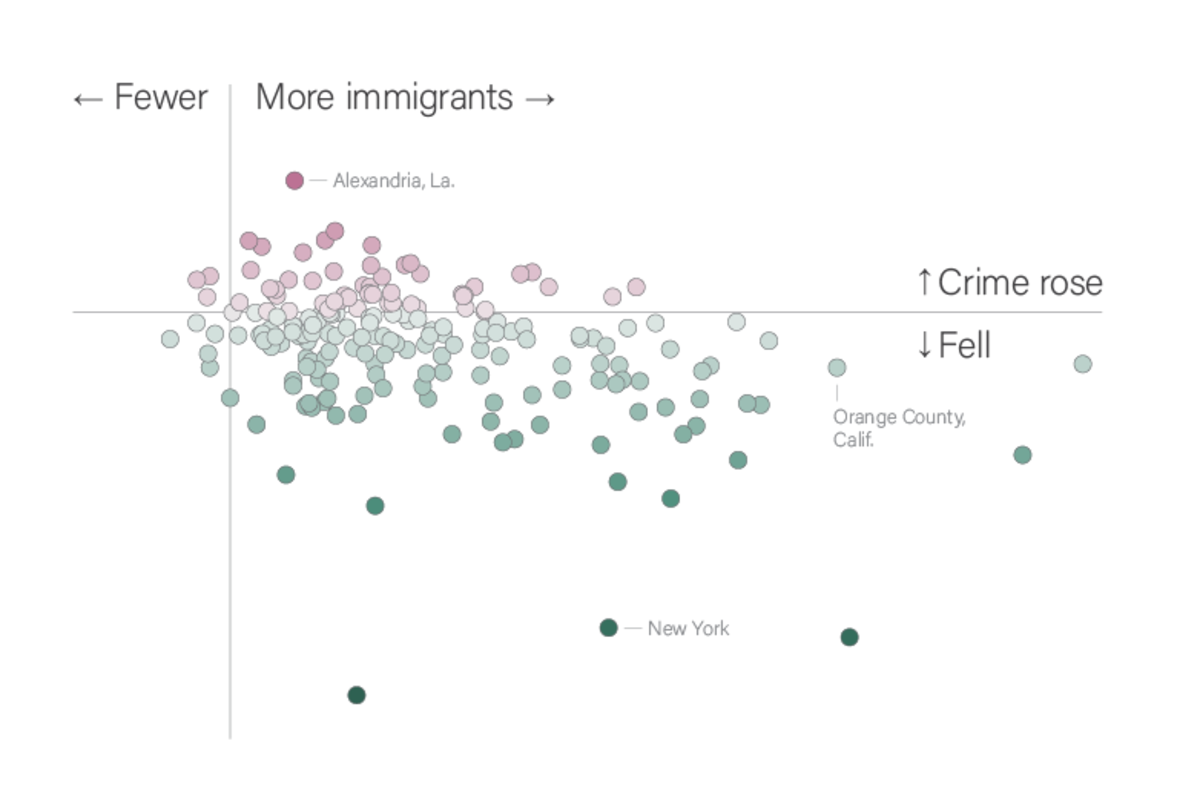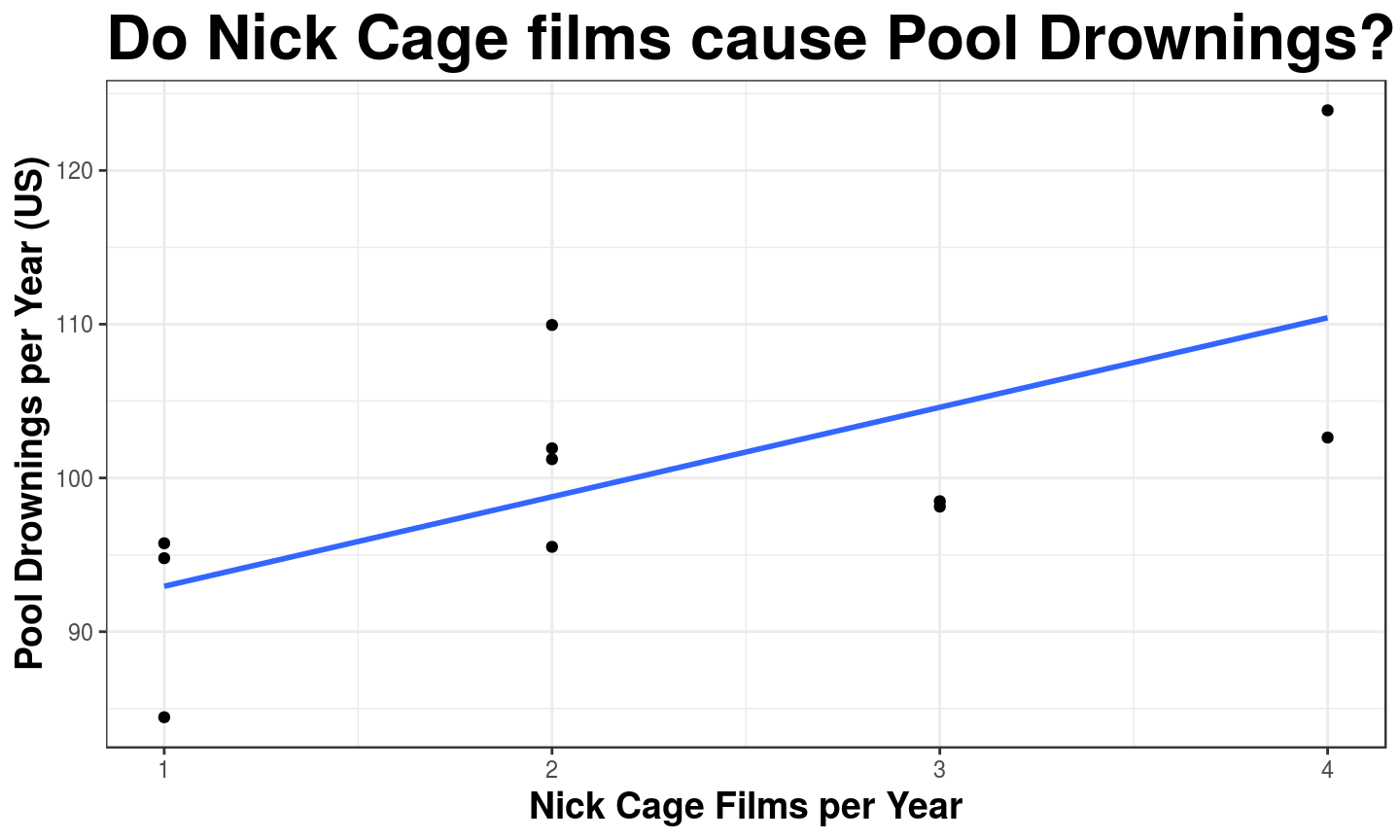March 6, 2019

## Plan for Today:

### (1) Causal Theories

• Causal Logics
• Independent/Dependent Variables

### (2) Testing Causal Theories

• hypotheses/empirical predictions
• fundamental problem of causal inference

## Causal Theory

To evaluate causal claims, we turn them into causal theories

A causal theory identifies systematic/structural causes that operate across space and time

• It is a kind of general knowledge
• Patterns/regularities within complexity
• and it is testable

## Causal Logics

One part of a causal theory is:

### causal logic:

Is a set of statements about how and why a cause $$C$$ produces its (claimed) effect $$E$$.

• causal chain connects cause $$C$$ to the effect $$E$$: $$C \xrightarrow{} e_1 \xrightarrow{} e_2 \xrightarrow{} e_3 \xrightarrow{} E$$
• Each step/mechanism is causal: $$e_1$$ is a cause of $$e_2$$

Assumptions/assertions

• Each step in the logic assumes a theory of how the world works/might work (why $$e_1 \to e_2$$)

## Making a causal logic

Causal logic for claim that $$C \xrightarrow{causes} E$$:

1. Must start with cause $$C$$, end with the effect $$E$$
2. Each step is related to the next through a causal relationship
3. Not a chronological list of specific events. It is a general sequence through which one things causes another (structural causes)

## Why causal logics?

1. Tell us how and when cause creates effect
• this can help refine the scope (generality) of the causal claim
2. Help us test causal claim
• we can test the main cause/effect, but also each step in the mechanism
3. Suggest other causes
• If $$C_1 \to e_1 \to e_2 \to E$$, then $$C_2$$ might $$\to e_2 \to E$$
4. Improve prescriptive claims
• If we can't change $$C$$, can we change $$e_2$$?
• Policies require specifics: causal logics more specific on how

## Causal Logics and "Scope"

"Increasing wealth causes countries to be less likely to experience civil war."

Under what conditions?

### A causal logic

1. Wealth $$\xrightarrow{}$$ Larger defense budget $$\xrightarrow{}$$ Rivals deterred $$\xrightarrow{}$$ Less civil war

## Causal Logics and "Testing"

"Increasing wealth causes countries to be less likely to experience civil war."

What tests are there?

### A different causal logic

1. Wealth $$\xrightarrow{}$$ Higher standard of living $$\xrightarrow{}$$ Fewer grievances $$\xrightarrow{}$$ Weak support for change $$\xrightarrow{}$$ Less civil war

## Causal Logics and "What to do"

You are a rebel (trying to start a civil war). You can't change the wealth of the country.

What would you do if 1 were true? 2? 3?

### Three competing causal logics

1. Wealth $$\xrightarrow{}$$ Larger defense budget $$\xrightarrow{}$$ Rivals deterred $$\xrightarrow{}$$ Less civil war
2. Wealth $$\xrightarrow{}$$ Higher standard of living $$\xrightarrow{}$$ Fewer grievances $$\xrightarrow{}$$ Weak support for change $$\xrightarrow{}$$ Less civil war
3. Wealth $$\xrightarrow{}$$ Lots of job opportunities $$\xrightarrow{}$$ Fewer listless young men $$\xrightarrow{}$$ Fewer rebel recruits $$\xrightarrow{}$$ Less civil war

## Causal Logics and "What to do"

You are the ruler (trying to prevent a civil war). You can't change the wealth of the country.

What would you do if 1 were true? 2? 3?

### Three competing causal logics

1. Wealth $$\xrightarrow{}$$ Larger defense budget $$\xrightarrow{}$$ Rivals deterred $$\xrightarrow{}$$ Less civil war
2. Wealth $$\xrightarrow{}$$ Higher standard of living $$\xrightarrow{}$$ Fewer grievances $$\xrightarrow{}$$ Weak support for change $$\xrightarrow{}$$ Less civil war
3. Wealth $$\xrightarrow{}$$ Lots of job opportunities $$\xrightarrow{}$$ Fewer listless young men $$\xrightarrow{}$$ Fewer rebel recruits $$\xrightarrow{}$$ Less civil war

## Causal Theories:

To turn a causal claim into a testable causal theory, we need:

1. causal logic connecting cause to effect: ✓
2. a statement of the direction of the effect: ✓
• can't just say "$$C$$ affects $$E$$" without saying whether it causes it to/to not happen; more or less of something.
3. restate causal claim in terms of what is observable:
• turn concepts into variables (exactly like with descriptive claims)
• independent variables
• dependent variables

## Variables and Causal Claims:

### Independent variable:

The variable capturing the purported cause in a causal claim.

• often denoted as "IV" or "$$X$$" or "right-hand variable"

### Dependent variable:

The variable capturing the purported outcome (what is affected) in a causal claim.

• often denoted as "DV" or "$$Y$$" or "left-hand variable"

### Why these terms?

Statistical/Mathematical expression: $$Y = f(X)$$ or $$DV = f(IV)$$

## Variables and Causal Claims:

### Causal Claim:

"Guns don't kill people, people kill people"

Which implies: "Increasing the prevalence of firearms in a region causes no change in human mortality"

How would you turn this claim into something observable?

Independent Variable ($$X$$): ?

Dependent Variable ($$Y$$): ?

## Variables and Causal Claims:

### Causal Claim:

"Exposure to anti-immigrant rhetoric on social media causes an increase in violence against immigrants"

Independent Variable ($$X$$): ?

Dependent Variable ($$Y$$): ?

## Causal Theories:

To turn a causal claim into a testable causal theory, we need:

1. causal logic connecting cause to effect: ✓
2. a statement of the direction of the effect: ✓
• can't just say "$$C$$ affects $$E$$" without saying whether it causes it to/to not happen; more or less of something.
3. restate causal claim in terms of what is observable: ✓
• turn concepts into variables (exactly like with descriptive claims)
• independent variables
• dependent variables

## Testing!

We turn causal claims into causal theories which are testable. We test causal theories by making…

### hypotheses/empirical predictions

these are statements about what we should observe if the causal claim is true.

• multiple hypotheses based on the overall causal claim and the causal logic
• Hypotheses state relationships between variables
• When $$X$$ is present(absent), $$Y$$ is present(absent)
• When $$X$$ is present(absent), $$Y$$ is more(less) likely
• When $$X$$ increases(decreases), $$Y$$ increases(decreases)

## Examples:

claim: "Ownership of firearms by citizens reduces crime"

independent variable: fraction of citizens owning firearms

dependent variable: crime victimization rate per 100 thousand

What is an empirical prediction/hypothesis?

## Examples:## Examples:

claim: "Increased immigration causes more crime"

independent variable: immigrant share of population

dependent variable: crime victimization rate per 100 thousand

What is an empirical prediction/hypothesis?

## Examples:## What are we missing?

### Causality is Counterfactual

If $$X$$ had been different for the same case and the same time, $$Y$$ would have been different

#### hypotheses from causal theories must be about potential outcomes

• recall: potential outcomes are values the dependent variable $$(Y)$$ would take for a case under different values of the independent variable $$(X)$$

## New hypotheses:

### Restate hypotheses in terms of counterfactuals

If there were more guns (in a specific place,time), there would have been less crime

If there were fewer immigrants (in a specific place, time), there would have been less crime

If there were fewer Nick Cage films release (in a country, year), there would have been fewer pool drownings

## A big problem

Hypotheses are empirical predictions: they are about what we should observe if $$X$$ causes $$Y$$.

The counterfactual approach to causality means: if $$X$$ causes $$Y$$ in some specific case, the potential outcomes of $$Y$$ are different across levels of $$X$$.

But for each case, only one potential outcome becomes "factual" and observable, the other(s) are counterfactual and unobservable